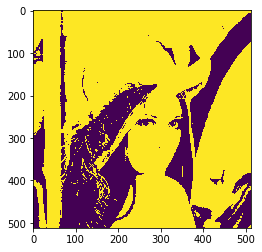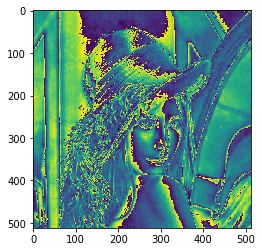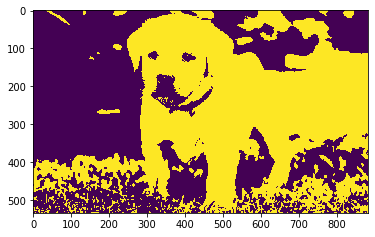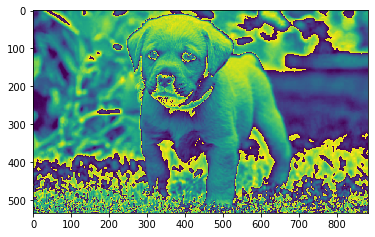Related Articles

# Mahotas – Conditional Eroding Image

• Last Updated : 27 Sep, 2021

In this article we will see how we can do conditional eroding of the image in mahotas. Erosion is one of two fundamental operations (the other being dilation) in morphological image processing from which all other morphological operations are based. It was originally defined for binary images, later being extended to grayscale images, and subsequently to complete lattices.

In this tutorial we will use “lena” image, below is the command to load it.

`mahotas.demos.load('lena')`

Below is the lena imageIn order to do this we will use mahotas.cerode method
Syntax : mahotas.cerode(img, c_grey)
Argument : It takes image object, conditional image as argument
Return : It returns image object

Note : Input image should be filtered or should be loaded as grey

In order to filter the image we will take the image object which is numpy.ndarray and filter it with the help of indexing, below is the command to do this

`image = image[:, :, 0]`

Below is the implementation

## Python3

 `# importing required libraries``import` `mahotas``import` `mahotas.demos``from` `pylab ``import` `gray, imshow, show``import` `numpy as np``  ` `# loading image``img ``=` `mahotas.demos.load(``'lena'``)`` ` `# grey image``g ``=` `img[:, :, ``1``]`` ` `# multiplying grey image values``g ``=` `g ``*` `3` `# filtering image``img ``=` `img.``max``(``2``)``  ` `# otsu method``T_otsu ``=` `mahotas.otsu(img)  ``  ` `# image values should be greater than otsu value``img ``=` `img > T_otsu``  ` `print``(``"Image threshold using Otsu Method"``)``  ` `# showing image``imshow(img)``show()``  ` `# eroding image using conditional grey image``new_img ``=` `mahotas.cerode(img, g)``  ` `# showing eroded image``print``(``"Eroded Image"``)``imshow(new_img)``show()`

Output :

`Image threshold using Otsu Method``Eroded Image`Another example

## Python3

 `# importing required libraries``import` `mahotas``import` `numpy as np``from` `pylab ``import` `gray, imshow, show``import` `os`` ` `# loading image``img ``=` `mahotas.imread(``'dog_image.png'``)` `# getting grey image``g ``=` `img[:, :, ``0``]` ` ` `# multiplying grey image values``g ``=` `g ``*` `2` `# filtering image``img ``=` `img[:, :, ``0``]``  ` `# otsu method``T_otsu ``=` `mahotas.otsu(img)  ``  ` `# image values should be greater than otsu value``img ``=` `img > T_otsu``  ` `print``(``"Image threshold using Otsu Method"``)``  ` `# showing image``imshow(img)``show()``  ` `# eroding image using conditional grey image``new_img ``=` `mahotas.cerode(img, g)``  ` `# showing eroded image``print``(``"Eroded Image"``)``imshow(new_img)``show()`

Output :

`Image threshold using Otsu Method``Eroded Image`Attention geek! Strengthen your foundations with the Python Programming Foundation Course and learn the basics.

To begin with, your interview preparations Enhance your Data Structures concepts with the Python DS Course. And to begin with your Machine Learning Journey, join the Machine Learning – Basic Level Course

My Personal Notes arrow_drop_up